# Lewis Symbols

We use Lewis symbols to describe valence electron configurations of atoms and monatomic ions. A Lewis symbol consists of an elemental symbol surrounded by one dot for each of its valence electrons: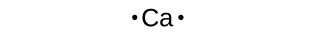Lewis symbols for the elements of the third period of the periodic table.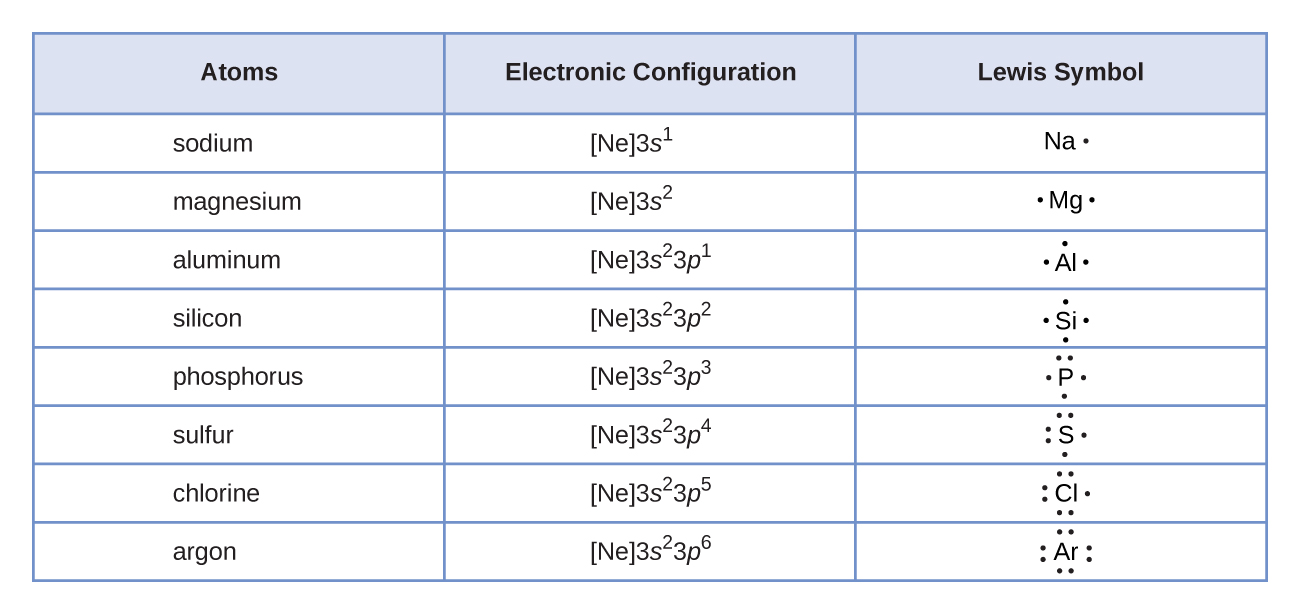Lewis symbols illustrating the number of valence electrons for each element in the third period of the periodic table.

Lewis symbols can also be used to illustrate the formation of cations from atoms, as shown here for sodium and calcium:Likewise, they can be used to show the formation of anions from atoms, as shown here for chlorine and sulfur:demonstrates the use of Lewis symbols to show the transfer of electrons during the formation of ionic compounds.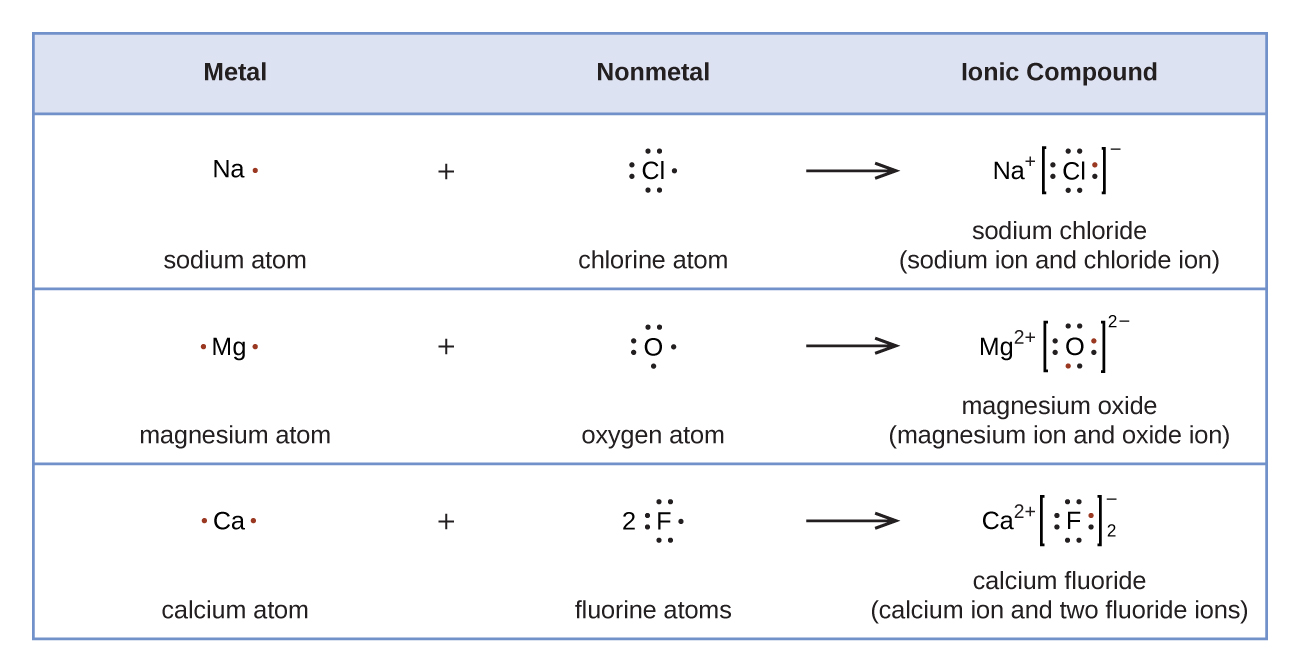Cations are formed when atoms lose electrons, represented by fewer Lewis dots, whereas anions are formed by atoms gaining electrons. The total number of electrons does not change.

# Lewis Structures

We also use Lewis symbols to indicate the formation of covalent bonds, which are shown in Lewis structures, drawings that describe the bonding in molecules and polyatomic ions. For example, when two chlorine atoms form a chlorine molecule, they share one pair of electrons: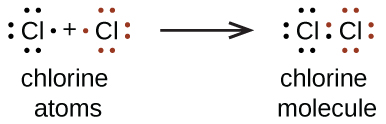The Lewis structure indicates that each Cl atom has three pairs of electrons that are not used in bonding (called lone pairs) and one shared pair of electrons (written between the atoms). A dash (or line) is sometimes used to indicate a shared pair of electrons:A single shared pair of electrons is called a single bond. Each Cl atom interacts with eight valence electrons: the six in the lone pairs and the two in the single bond.

## The Octet Rule

The other halogen molecules (F2, Br2, I2, and At2) form bonds like those in the chlorine molecule: one single bond between atoms and three lone pairs of electrons per atom. This allows each halogen atom to have a noble gas electron configuration. The tendency of main group atoms to form enough bonds to obtain eight valence electrons is known as the octet rule.The Octet Rule. For example , fluorine has an electron configuration notation of. 1s2, 2s2, 2p5. In fluorine’s highest occupied energy level it has 2 electrons in the s sublevel and 5 electrons in the p sublevel. The total number of electrons in fluorine’s highest occupied energy level is therefore = 7. One fluorine atoms bonds to a second fluorine atom to form F2 (fluorine gas, a compound). Each atom shares one of its valence electrons with its partner to get 8 electrons in the valence shell.

The number of bonds that an atom can form can often be predicted from the number of electrons needed to reach an octet (eight valence electrons); this is especially true of the nonmetals of the second period of the periodic table (C, N, O, and F). For example, each atom of a group 14 element has four electrons in its outermost shell and therefore requires four more electrons to reach an octet.

These four electrons can be gained by forming four covalent bonds, as illustrated here for carbon in CCl4 (carbon tetrachloride) and silicon in SiH4 (silane). Because hydrogen only needs two electrons to fill its valence shell, it is an exception to the octet rule. The transition elements and inner transition elements also do not follow the octet rule: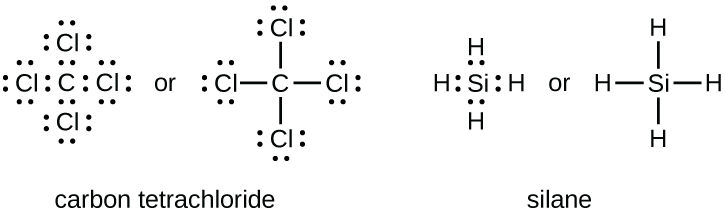Group 15 elements such as nitrogen have five valence electrons in the atomic Lewis symbol: one lone pair and three unpaired electrons. To obtain an octet, these atoms form three covalent bonds, as in NH3 (ammonia). Oxygen and other atoms in group 16 obtain an octet by forming two covalent bonds: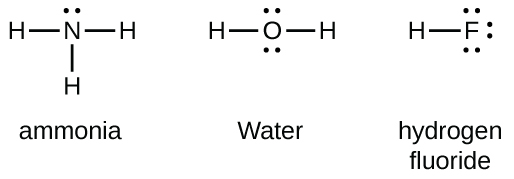## Double and Triple Bonds

As previously mentioned, when a pair of atoms shares one pair of electrons, we call this a single bond. However, a pair of atoms may need to share more than one pair of electrons in order to achieve the requisite octet. A double bond forms when two pairs of electrons are shared between a pair of atoms, as between the carbon and oxygen atoms in CH2O (formaldehyde) and between the two carbon atoms in C2H4 (ethylene):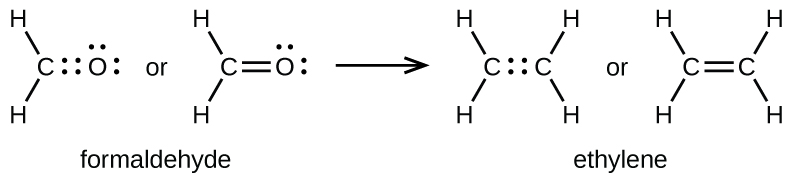triple bond forms when three electron pairs are shared by a pair of atoms, as in carbon monoxide (CO) and the cyanide ion (CN):# Writing Lewis Structures with the Octet Rule

For very simple molecules and molecular ions, we can write the Lewis structures by merely pairing up the unpaired electrons on the constituent atoms. See these examples: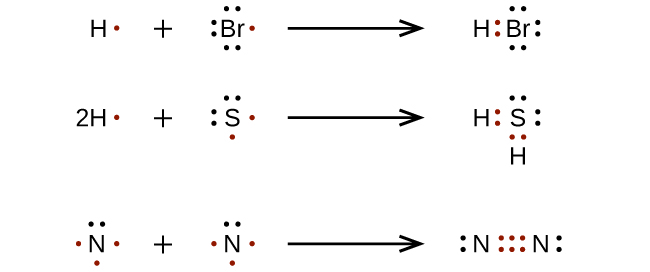For more complicated molecules and molecular ions, it is helpful to follow the step-by-step procedure outlined here:

1. Determine the total number of valence (outer shell) electrons. For cations, subtract one electron for each positive charge. For anions, add one electron for each negative charge.
2. Draw a skeleton structure of the molecule or ion, arranging the atoms around a central atom. (Generally, the least electronegative element should be placed in the center.) Connect each atom to the central atom with a single bond (one electron pair).
3. Distribute the remaining electrons as lone pairs on the terminal atoms (except hydrogen), completing an octet around each atom.
4. Place all remaining electrons on the central atom.
5. Rearrange the electrons of the outer atoms to make multiple bonds with the central atom in order to obtain octets wherever possible.

Let us determine the Lewis structures of SiH4, CHO2−, NO+, and OF2 as examples in following this procedure:

1. Determine the total number of valence (outer shell) electrons in the molecule or ion.
• For a molecule, we add the number of valence electrons on each atom in the molecule:$\begin{array}{r r l} \text{SiH}_4 & & \\[1em] & \text{Si: 4 valence electrons/atom} \times 1 \;\text{atom} & = 4 \\[1em] \rule[-0.5ex]{21em}{0.1ex}\hspace{-21em} + & \text{H: 1 valence electron/atom} \times 4 \;\text{atoms} & = 4 \\[1em] & & = 8 \;\text{valence electrons} \end{array}$
• For a negative ion, such as CHO2, we add the number of valence electrons on the atoms to the number of negative charges on the ion (one electron is gained for each single negative charge):$\begin{array}{r r l} {\text{CHO}_2}^{-} & & \\[1em] & \text{C: 4 valence electrons/atom} \times 1 \;\text{atom} & = 4 \\[1em] & \text{H: 1 valence electron/atom} \times 1 \;\text{atom} & = 1 \\[1em] & \text{O: 6 valence electrons/atom} \times 2 \;\text{atoms} & = 12 \\[1em] \rule[-0.5ex]{21.5em}{0.1ex}\hspace{-21.5em} + & 1\;\text{additional electron} & = 1 \\[1em] & & = 18 \;\text{valence electrons} \end{array}$
• For a positive ion, such as NO+, we add the number of valence electrons on the atoms in the ion and then subtract the number of positive charges on the ion (one electron is lost for each single positive charge) from the total number of valence electrons:$\begin{array}{r r l} \text{NO}^{+} & & \\[1em] & \text{N: 5 valence electrons/atom} \times 1 \;\text{atom} & = 5 \\[1em] & \text{O: 6 valence electrons/atom} \times 1 \;\text{atom} & = 6 \\[1em] \rule[-0.5ex]{21em}{0.1ex}\hspace{-21em} + & -1 \;\text{electron (positive charge)} & = -1 \\[1em] & & = 10 \;\text{valence electrons} \end{array}$
• Since OF2 is a neutral molecule, we simply add the number of valence electrons:$\begin{array}{r r l} \text{OF}_{2} & & \\[1em] & \text{O: 6 valence electrons/atom} \times 1 \;\text{atom} & = 6 \\[1em] \rule[-0.5ex]{21em}{0.1ex}\hspace{-21em} + & \text{F: 7 valence electrons/atom} \times 2 \;\text{atoms} & = 14 \\[1em] & & = 20 \;\text{valence electrons} \end{array}$
2. Draw a skeleton structure of the molecule or ion, arranging the atoms around a central atom and connecting each atom to the central atom with a single (one electron pair) bond. (Note that we denote ions with brackets around the structure, indicating the charge outside the brackets:)When several arrangements of atoms are possible, as for CHO2, we must use experimental evidence to choose the correct one. In general, the less electronegative elements are more likely to be central atoms. In CHO2, the less electronegative carbon atom occupies the central position with the oxygen and hydrogen atoms surrounding it. Other examples include P in POCl3, S in SO2, and Cl in ClO4. An exception is that hydrogen is almost never a central atom. As the most electronegative element, fluorine also cannot be a central atom.
3. Distribute the remaining electrons as lone pairs on the terminal atoms (except hydrogen) to complete their valence shells with an octet of electrons.
• There are no remaining electrons on SiH4, so it is unchanged: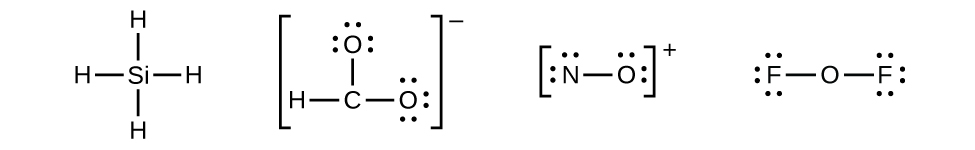4. Place all remaining electrons on the central atom.
• For SiH4, CHO2, and NO+, there are no remaining electrons; we already placed all of the electrons determined in Step 1.
• For OF2, we had 16 electrons remaining in Step 3, and we placed 12, leaving 4 to be placed on the central atom: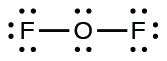5. Rearrange the electrons of the outer atoms to make multiple bonds with the central atom in order to obtain octets wherever possible.
• SiH4: Si already has an octet, so nothing needs to be done.
• CHO2: We have distributed the valence electrons as lone pairs on the oxygen atoms, but the carbon atom lacks an octet:• NO+: For this ion, we added eight valence electrons, but neither atom has an octet. We cannot add any more electrons since we have already used the total that we found in Step 1, so we must move electrons to form a multiple bond:This still does not produce an octet, so we must move another pair, forming a triple bond:• In OF2, each atom has an octet as drawn, so nothing changes.

IMPORTANT : no Lewis diagram is complete without formal charges.  Lewis diagrams are drawn to examine mechanisms so knowing which parts of a molecule are electron defficient (+) and which are electron rich (-) is vital.
It is best to have a formal charge of 0 for as many of the atoms in a structure as possible.
If a formal charge of 1- is located next to a formal charge of 1+, the formal charges can usually be minimized by having a lone pair of electrons, located on the atom with the 1- charge become a bonding pair of electrons that is shared with the atom that has the 1+ formal charge (this can be visualised in the same way as the formation of multiple bonds were above).

CAUTION : octets can be expanded to minimise formal charges but only for atoms in the second row of the periodic table  (where n=3 or greater).   For instance in our example, N cannot expand its octet so keeps a formal charge of 1+ and both singly bonded oxygens a formal charge of 1-.  If our molecule were SO3 , however, it would be possible to minimize all formal charges by having the sulfur expand its octet.

LEARNING ABOUT THE DRAWING OF LEWIS STRUCTURE

## STEM Elearning

We at FAWE have built this platform to aid learners, trainers and mentors get practical help with content, an interactive platform and tools to power their teaching and learning of STEM subjects, more

#### How to find your voice as a woman in Africa

© FAWE, Powered by: Yaaka DN.